# Free Candy Subtraction Cut & Paste Worksheet

You can have fun matching subtraction equations and answers with the candy jar subtraction cut and paste.

A simple subtraction worksheet that is great for learning in first grade and second grade review of subtraction.

You can solve color, cut, and paste the answers.

The Free Candy Subtraction Cut & Paste Printable is part of the 20 Free printables for October.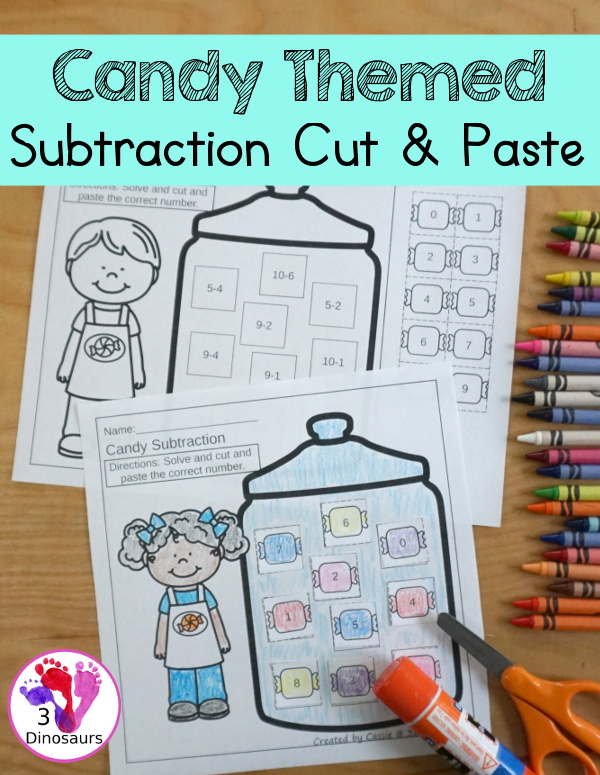We have included links to some of the products and resources we have used with this activity. If you purchase via the links we may earn a small commission.

This is a great addition to the subtraction prinatbles and Halloween Printables on the site.

## What is Subtraction?

Subtraction is the removal of objects from a collection. So you start with three objects and take one of the objects away and you have two remaining.

The equation for subtraction would look like 3 – 1 = 2.

The candy subtraction printable works on subtracting with 10 different equations that only have one possible answer. You have 0 to 9 for the answers.## Things to use with the Candy Subtraction Cut & Paste Worksheet Printable

There are all the items we used with this post. You can check out all the links below on Amazon to make it easy to find the items.

• printer• white copy paper• crayons
• scissors• glue stick## Ways to Use the Candy Subtraction Cut & Paste Worksheet Printable

You are going to see the steps below that we used with this candy subtraction cut and paste.

### Setting up the Subtraction Printable

You can see below how we set up the subtraction learning center. You have the subtraction worksheet, scissors, and glue stick. You can also include crayons and pencils.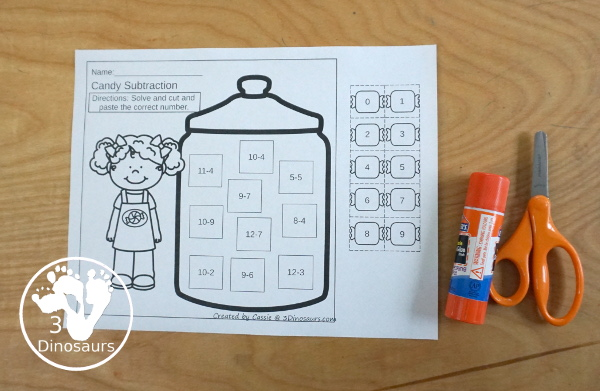### Cut the Answers off the Side

You can start by cutting off the answer on the side. You don’t have to do this first but it is easy to do at the start.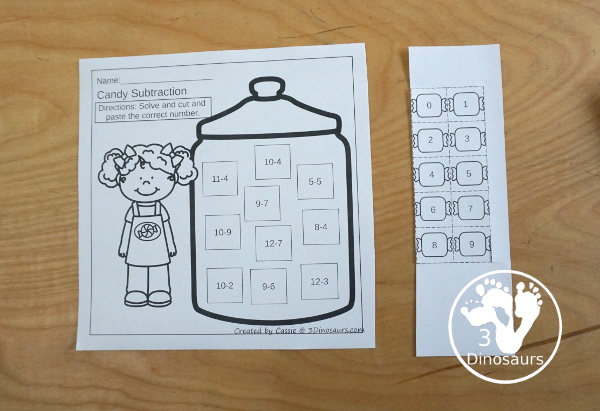### Match the Candy to the Correct Subtraction Equation

You solve the subtraction problems and then match the candy answers to the equations. You can write the answers to the equations below it to help match the numbers or just match the numbers.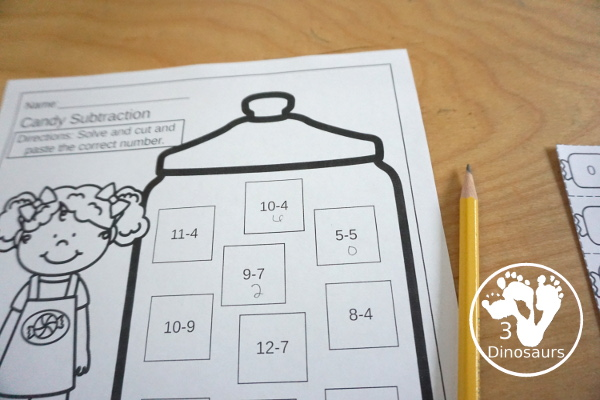Then paste the candy answers onto the subtraction equations.### Color the Candy Jar

After you finish you can color the candy jar. It is a fun way to see the finished math activity. Plus kids can enjoy doing subtraction math.## More Candy Themed Math Printables

If you are looking for more candy themed math you can check out the Candy No-Prep Addition and Subtraction Pack. It has 30 pages of candy themed math for kids to do.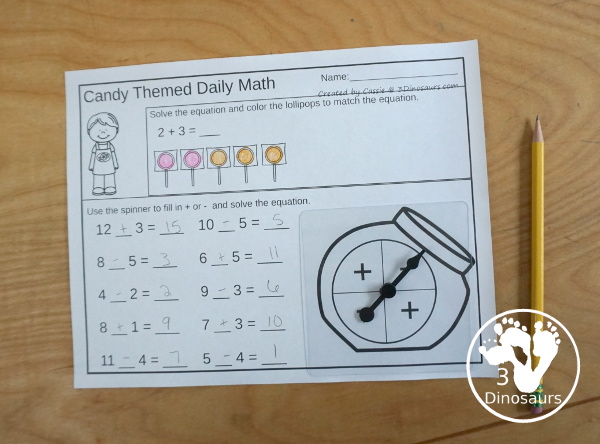## What in the No-Prep Candy Themed Math Printables Addition & Subtraction

It is a collection of 30 pages on no-prep activities for addition and subtraction. It also includes great math center activities of roll and graph and a math fact sheet.### What you will find in the Candy Themed Math Printables Addition & Subtraction Printables:

• 30 pages of no-prep printables for addition and subtraction
• Halloween Themed Math Fact Review Page
• Roll & Graph Addition for 1 to 6 and 1 to 10
• Spinner to use with Roll & Graph and math fact page
• Price: \$4

## What you will find in the Free Candy Subtraction Cut & Paste Worksheet

• 2 cut and paste subtraction worksheets
• Subtraction from 1 to 10
• Subtraction from 1 to 15
• 2 pages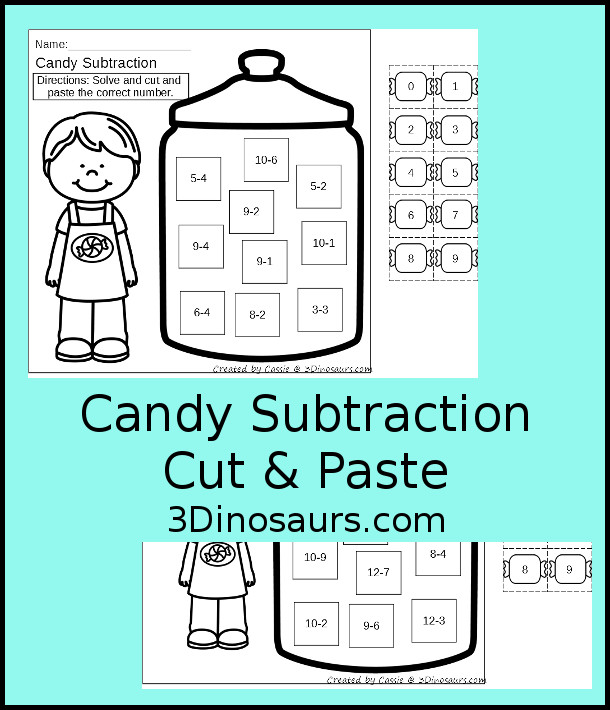## Check out these other Candy Math Printables

No-Prep Candy Weekly Packs – Prek to Fourth Grade a fun mix of math and language activities.

Roll a Candy Jar Math is a great hands-on math activity with counting, adding and multiplication.

No-Prep Candy Math Packs has 30 pages of no-prep candy themed math with addition and subtraction for kids to work on.

Candy Themed Skip Counting by 8 Puzzles is a fun puzzle set that kids can use to learn to skip counting!

Cassie – 3Dinosaurs.com